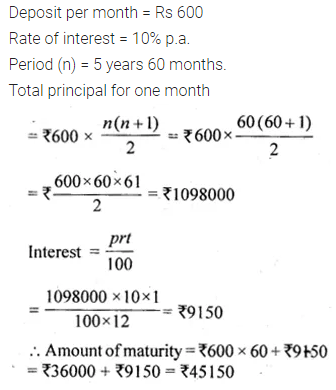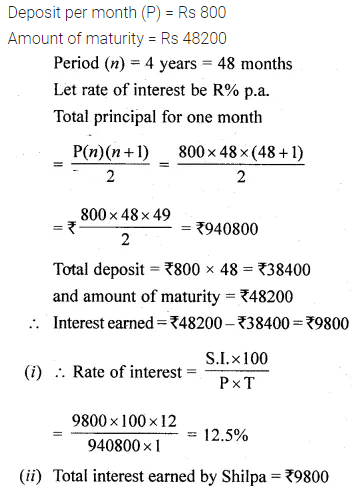# ML Aggarwal Class 10 Solutions for ICSE Maths Chapter 2 Banking Chapter Test

## ML Aggarwal Class 10 Solutions for ICSE Maths Chapter 2 Banking Chapter Test

ML Aggarwal Class 10 Solutions for ICSE Maths Chapter 2 Banking Chapter Test

Question 1.
Mr Dhruv deposits Rs 600 per month in a recurring deposit account for 5 years at the rate of 10% per annum (simple interest). Find the amount he will receive at the time of maturity.
Solution:Question 2.
Ankita started paying Rs 400 per month in a 3 years recurring deposit. After six months her brother Anshul started paying Rs 500 per month in a $$2 \frac { 1 }{ 2 }$$ years recurring deposit. The bank paid 10% p.a. simple interest for both. At maturity who will get more money and by how much?
Solution:Question 3.
Shilpa has a 4-year recurring deposit account in Bank of Maharashtra and deposits Rs 800 per month. If she gets Rs 48200 at the time of maturity, find
(i) the rate of simple interest,
(ii) the total interest earned by Shilpa
Solution:Question 4.
Mr. Chaturvedi has a recurring deposit account in Grindlay’s Bank for $$4 \frac { 1 }{ 2 }$$ years at 11% p.a. (simple interest). If he gets Rs 101418.75 at the time of maturity, find the monthly instalment.
Solution:Question 5.
Rajiv Bhardwaj has a recurring deposit account in a bank of Rs 600 per month. If the bank pays simple interest of 7% p.a. and he gets Rs 15450 as maturity amount, find the total time for which the account was held.
Solution: